# Hendecagons - polygons with 11 sides

By Martin McBride, 2022-10-10
Tags: hendecagon 11 sided shape regular polygon irregular polygon
Categories: gcse geometryA hendecagon is a flat shape with 11 straight sides.

## Regular and irregular hendecagons

A regular hendecagon is a 11-sided shape where every side is the same length and every corner has the same angle. All regular hendecagons have the same shape, like this: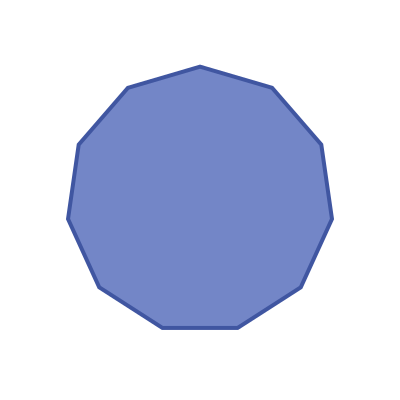An irregular hendecagon is a 11 sides shape where not every side and angle are equal. There are many different irregular hendecagon shapes, here is an example: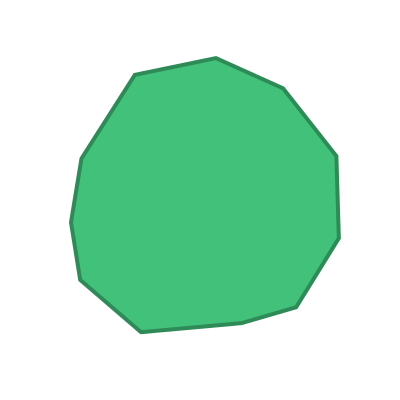## Name

The name hendecagon is a combination of the words hendeca (Greek meaning eleven) and gonia (Greek meaning corner).

Hendecagons are sometimes called 11-gons.

## Interior angles

The interior angles of a hendecagon are shown here:The sum of these 11 angles is given by the formula:

sum of interior angles = (n - 2) x 180


Where n is the number of sides. In this case, the number of sides n is 11, so the sum of the interior angles is:

(11 - 2) x 180 = 1620 degrees


For a regular hendecagon, all the interior angles are equal: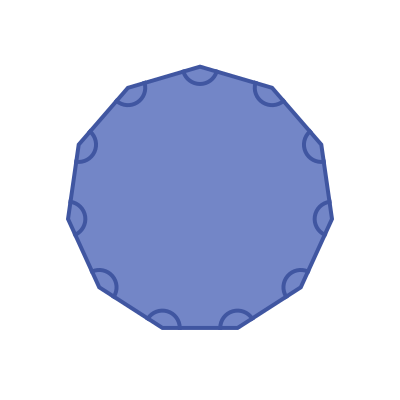This means that the interior angle of a regular hendecagon is:

1620 / 11 =  147.27 degrees (approximately)


## Exterior angles

The exterior angles of a hendecagon are shown here: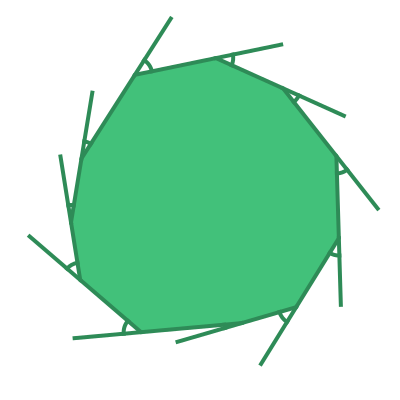The sum of the exterior angles of any polygon is 360 degrees.

For a regular hendecagon, all the interior angles are equal: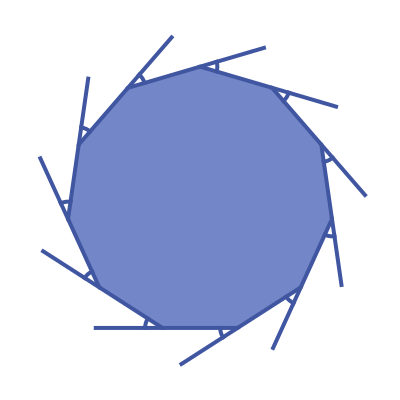This means that the exterior angle of a regular hendecagon is:

360 / 11 =  32.73 degrees (approximately)


## Symmetry of regular hendecagons

A regular hendecagon has 11 lines of symmetry. This means that it can be reflected over each of the 11 grey lines shown here: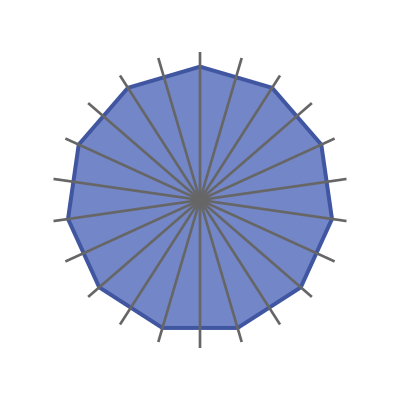A regular hendecagon has rotational symmetry of order 11. This means that if it rotated about its centre by a 11th of a full turn, it will map onto itself: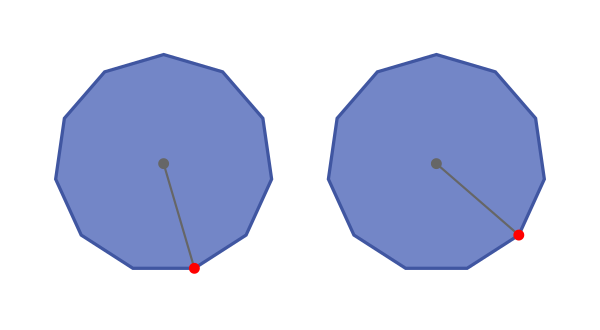## Real life examples

The Canadian 1 dollar coin is based on the regular hendecagon.